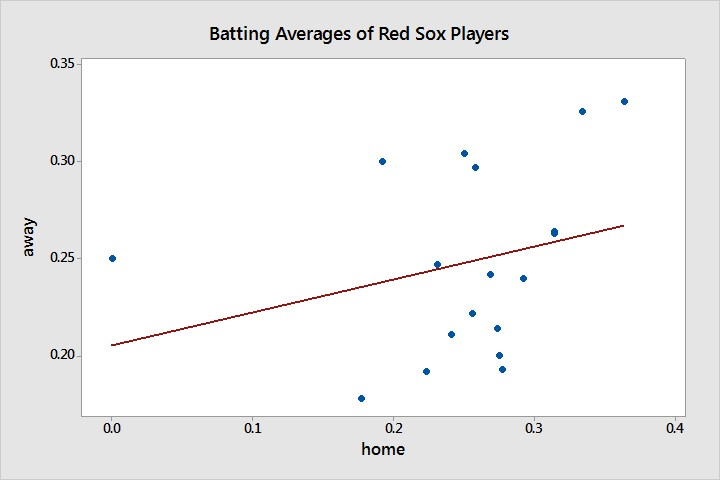# 7.1 - Scatterplots

7.1 - Scatterplots

With Clark’s interest in investigating the relationship between two quantitative variables, he correctly started with a scatterplot.

Scatterplot
A graphical representation of two quantitative variables where the explanatory variable is on the x-axis and the response variable is on the y-axis.

When we look at the scatterplot, keep in mind the following questions:

1. What is the direction of the relationship?
2. Is the relationship linear or nonlinear?
3. Is the relationship weak, moderate, or strong?
4. Are there any outliers or extreme values?

We describe the direction of the relationship as positive or negative. A positive relationship means that as the value of the explanatory variable increases, the value of the response variable increases, in general. A negative relationship implies that as the value of the explanatory variable increases, the value of the response variable tends to decrease.

Looking at Clark’s scatterplot, we see a positive direction. As home batting average is increasing in value (moving toward the right on the X axis) away batting average is also increasing in value (moving up the Y axis).While Clark does not have a lot of data, it is possible to see that the pattern of the data suggests a linear (straight line) pattern. There is no discernable curve to the pattern. While this is not as obvious as we might like it to be, for real-world data, this is pretty typical.

Finally, we want to as about the magnitude of the data. We do this by looking at the degree of the slope (think running up a hill). The slope of the red line indicates a slope that is closer to a zero line (no hill running) then a steep slope (hard hill running).

Now that Clark has taken a closer look at describing his data, let’s take a closer look at analyzing the relationship between home and away batting averages!

  Link ↥ Has Tooltip/Popover Toggleable Visibility